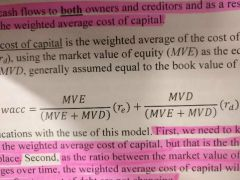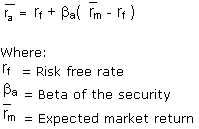• Shuffle
Toggle On
Toggle Off
• Alphabetize
Toggle On
Toggle Off
• Front First
Toggle On
Toggle Off
• Both Sides
Toggle On
Toggle Off
Toggle On
Toggle Off
Front

## Card Range To Study

throughPlay buttonPlay buttonProgress

1/19

Click to flip

Use LEFT and RIGHT arrow keys to navigate between flashcards;

Use UP and DOWN arrow keys to flip the card;

H to show hint;

 Formula for Big Dividend Beginning Owners Equity +Comprehensive Income - End Owners' Equity Formula for Estimated Value per Share Estimated Value /Shares Outstanding Formula for Dividend Discount Model Estimated Value at time zero = Forecasted dividend for period/ (1 + cost of equity capital)^period Formula for Book Value Book Value of owner's equity at time t = Book Value at time t-1 + Comprehensive Income - Big Dividend Dividend Discount Model The dividend discount model (DDM) is a method of valuing a company's stock price based on the theory that its stock is worth the sum of all of its future dividend payments, discounted back to their present value. "Big Dividends" Dividends used to value firms Book Value Book value of an asset is the value at which the asset is carried on a balance sheet and calculated by taking the cost of an asset minus the accumulated depreciation. Weighted average cost of capital is the average rate of return a company expects to compensate all its different investors. The weights are the fraction of each financing source in the company's target capital structure. Formula for weighted average cost of capitalFormula for Cash Flow Cash Flow = Big Dividend + After-tax Interest Expense - Change in Long Term Debt Cost of Equity Capital The return that investors expect to receive from an equity investment. Capital Asset Pricing Model - CAPM The capital asset pricing model (CAPM) is a model that describes the relationship between systematic risk and expected return for assets, particularly stocks. Formula for Capital Asset Pricing Model - CAPMWhat are "transitionary" components of income? Components of income not expected to recur. What are "persistent" components of income? Components of income which are expected to recur. What are Discontinued Operations When a company decides to sell or dispose of a part of its business and the disposal represents a strategic shift that has a major effect on an entities operations and financial results. Special Items A transaction or an event that is relatively unusual or expected to be non-recurring that affects net-imcome What are Restructurings A restructuring is a package of changes to the company's operations intended to improve efficiency and profitability. Pro Forma (Non - GAAP) Income Alternative Measures of income that don't abide by Generally Accepted Accounting Principles.